# Simple Relation Example

## Coincides

This is a synchronous temporal relation for which the nth tick of the left clock appears simultaneously with the nth tick of the right clock.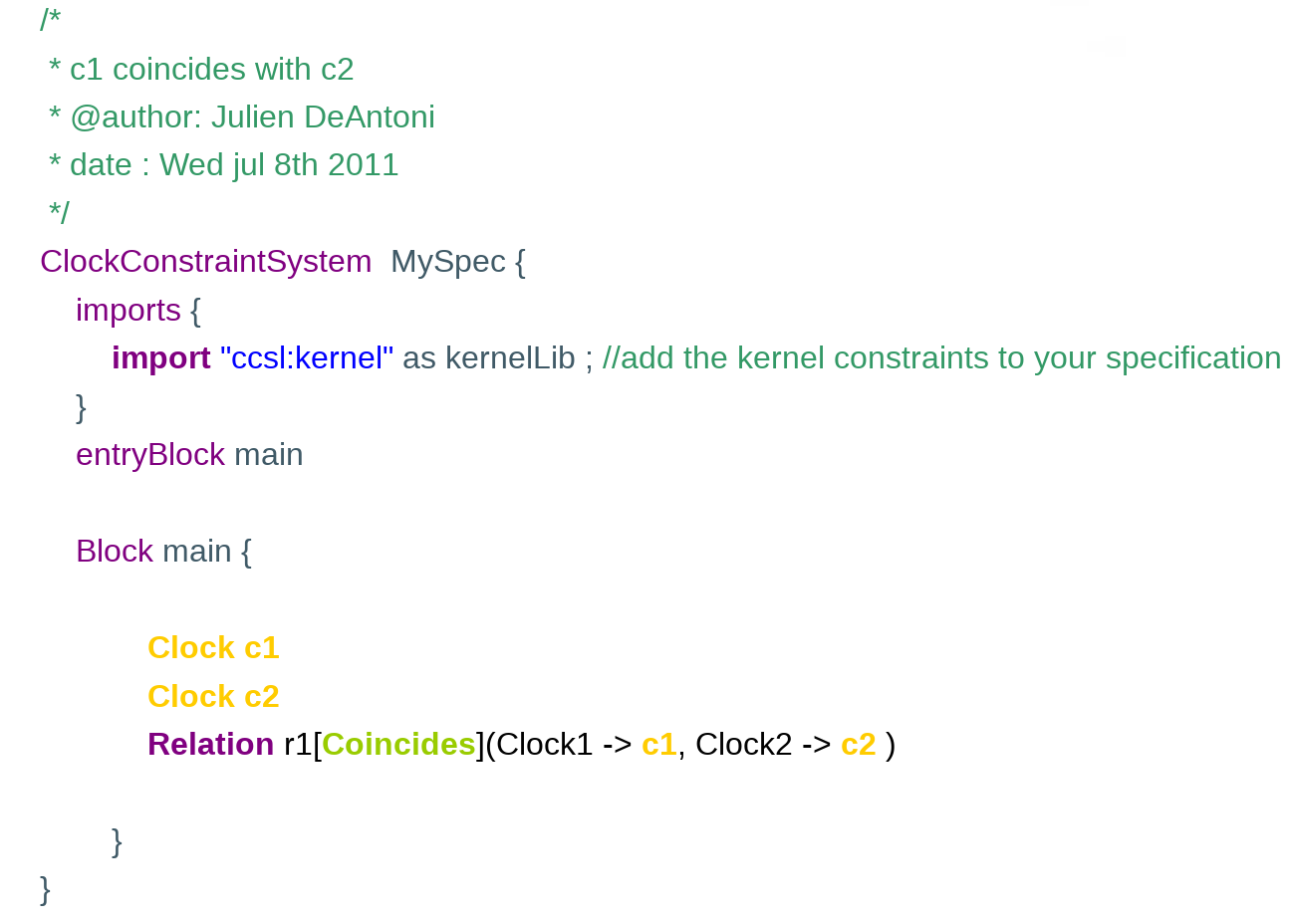Example of CCSL specification:

Simulation results:on the top, the timing diagram (VCD) where the pulses represent the ticks of a clock.

The horizontal axis represents the logical time increase.

On the left bottom, the constraint that can be represented on the timing diagram; the next picture shows the representation of the relation between the instants in this diagram. While the simulation is computed as a total order, the instant relation computed during the simulation (and represented on the next picture) provides one of the partial orderings that is an acceptable solution for the specification. Of course, with only a single constraint (coincides) the solution is unique and the order is total.

Finally, on the bottom right, the clock domains are represented; here the two clocks are linked by a synchronous relation: ==. Note that it does not mean that objects are equals; each clock has its own identity but the equality in time: they always tick simultaneously.

## Exclusion

This is a temporal relations that prevents two clocks to tick simultaneously.

Example of CCSL specification: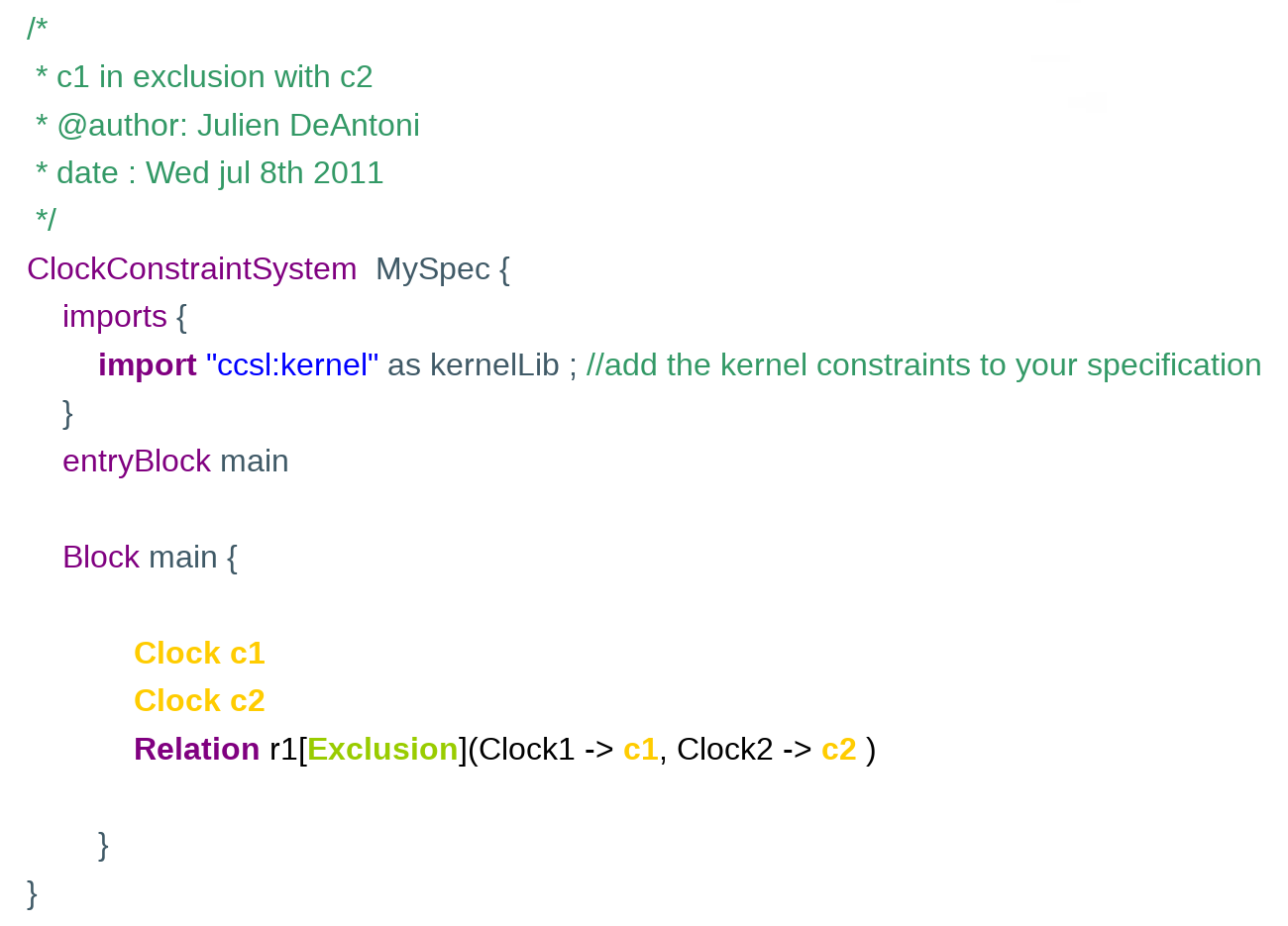Simulation results:On the bottom right, the clock domains are represented; here the two clocks are linked by an exclusion relation: #.

## SubClock

This is a synchronous temporal relation that prevents one clock (the subclock) from ticking when another master clock (the superclock) does not tick.

Example of CCSL specification: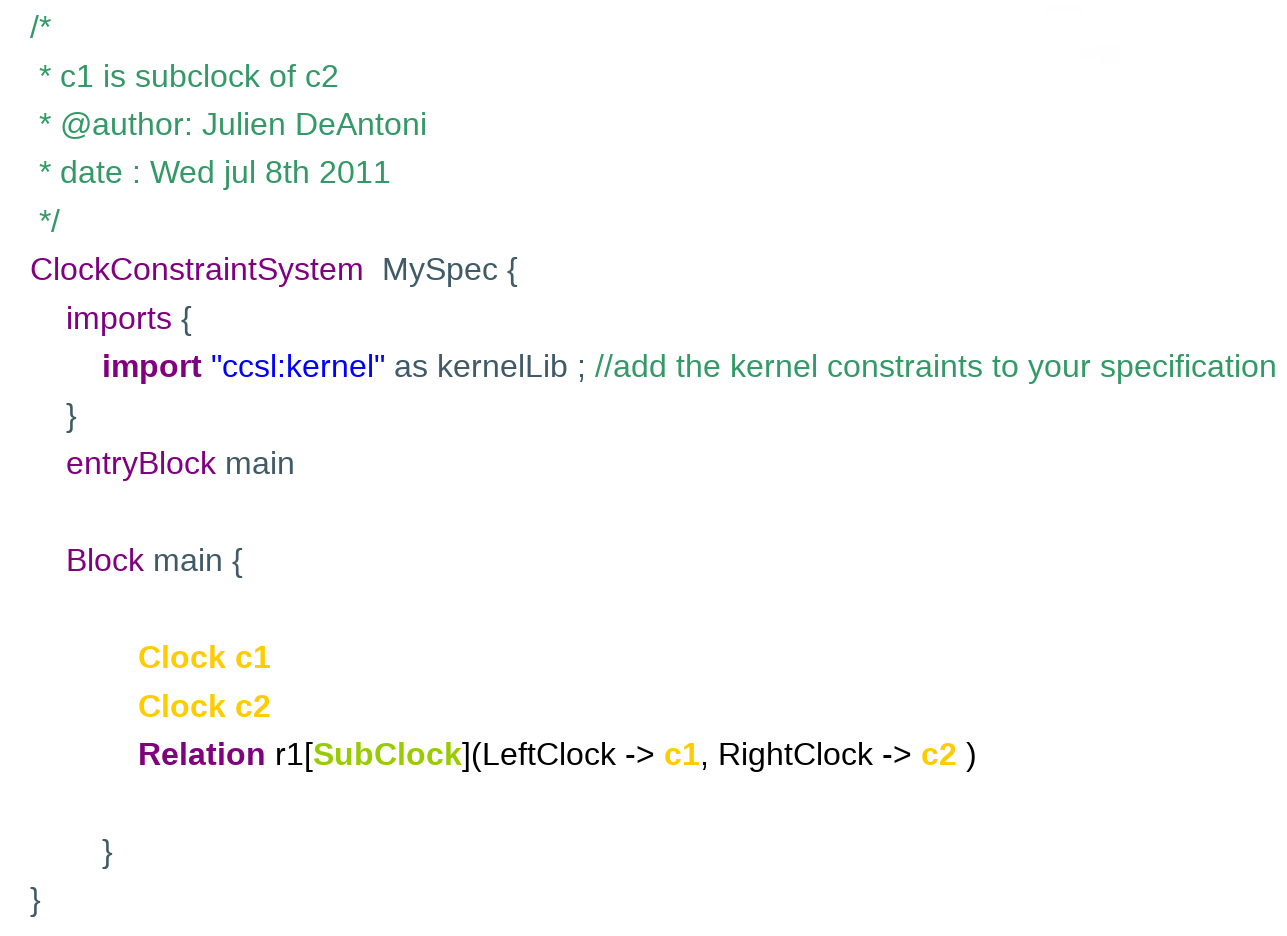Simulation results:

The subClock relation is simulated in the next picture. Some ticks of c2 occurs at the same time than a tick of c1.

On the bottom right, the clock domain is represented; here, the two clocks are linked by a subclock relation.## Precedes

This is an asynchronous temporal relation for which the nth tick of the left clock occurs before the nth tick of the right clock. In short, the left clock is faster than the right clock.

Example of a CCSL specification: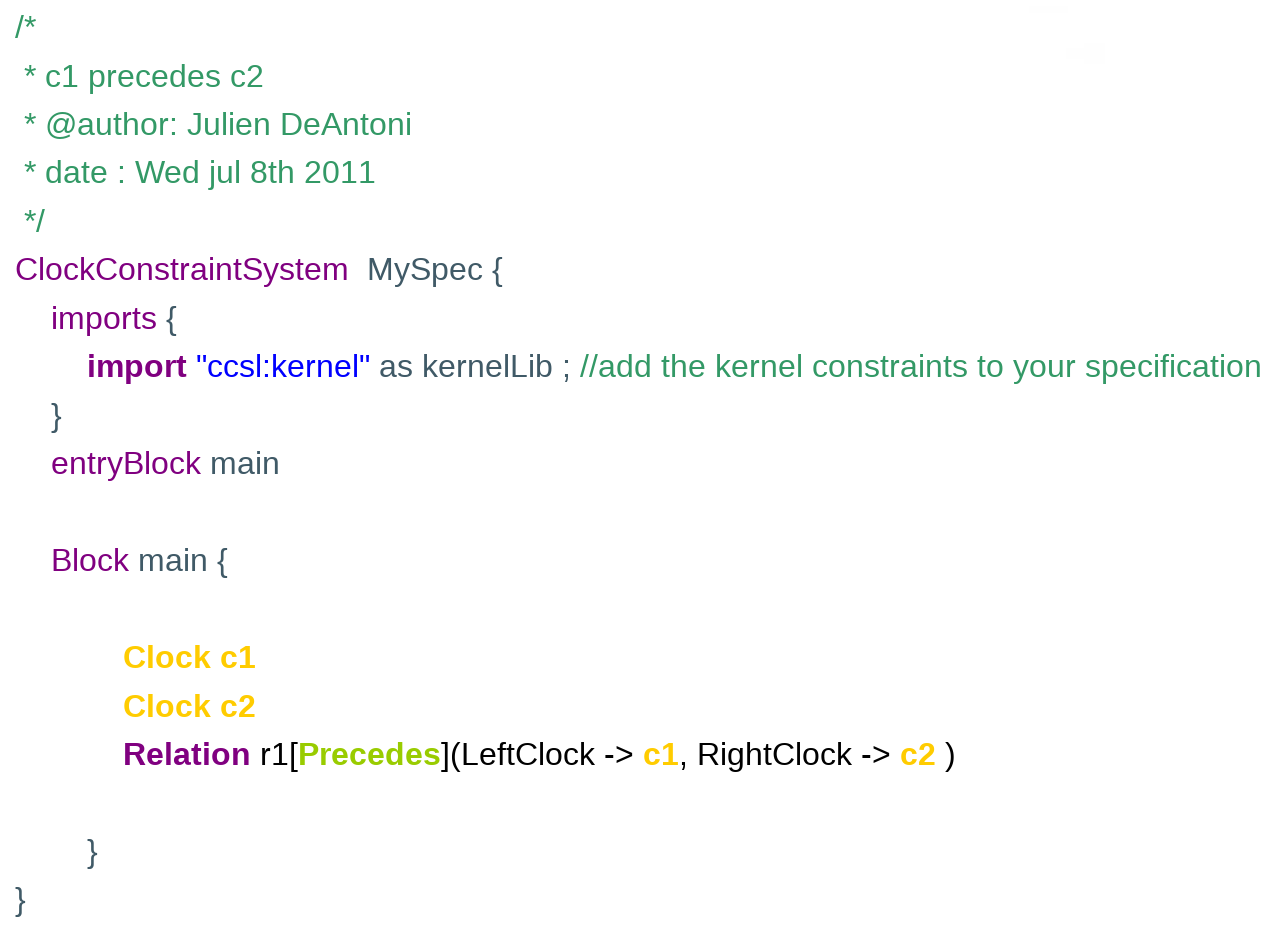Simulation results:on the top, the timing diagram (VCD) where the pulses represent the ticks of clocks.

The horizontal sequence represents the logical time increase.

On the left bottom, the constraint that can be represented on the timing diagram; the next picture shows the representation of the relation between the instants in this diagram. While the simulation is computed as a total order, the instant relations computed during the simulation (and represented on the next picture) provide one of the partial orders that are acceptable solutions of the specification.

Finally, on the bottom right, the clock domains are represented; here the two clocks are linked by an asynchronous relation: precedes. In the other examples, you could see the subclock / equality or exclusion relations.## Causes

This is a causality relation for which the nth tick of the left clock appears either before or at the same time than the nth tick of the right clock (the causality is not temporal but the effect cannot appear before the causes).

Example of a CCSL specification: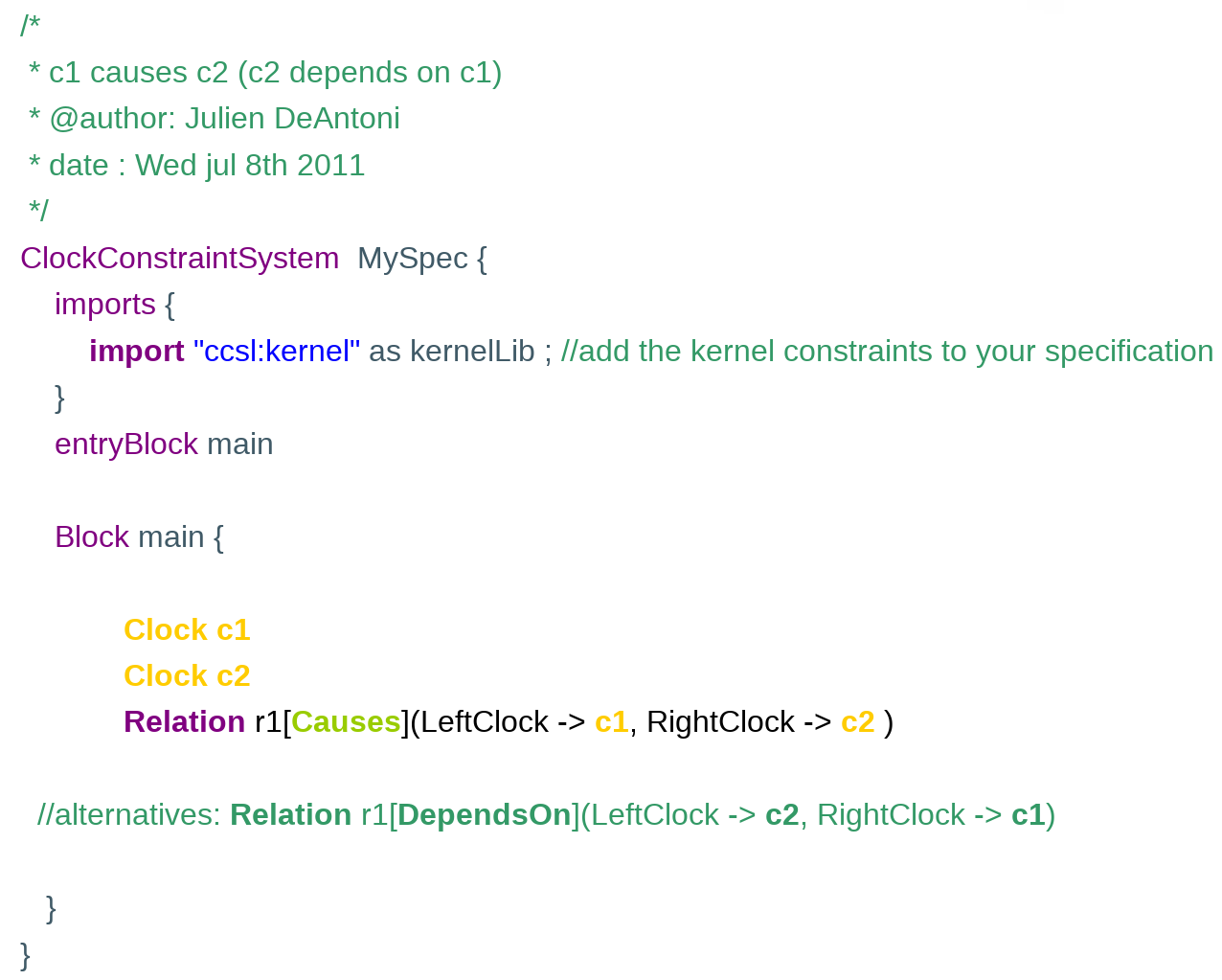Simulation results:

The Causes relation is simulated on the next picture. Some ticks of c2 occur after their corresponding tick in c1 and some others at the same time…
On the bottom right, the clock domain is represented; here, the two clocks are linked by an untimed relation (<= means either < or ==).